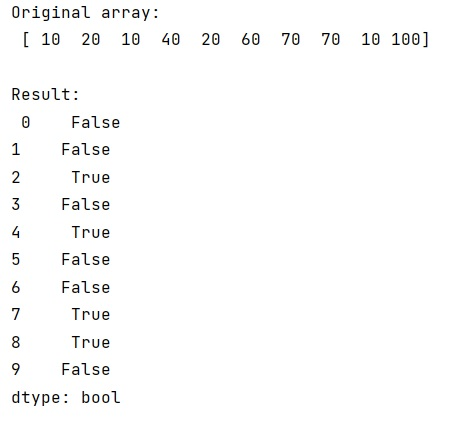# Determining duplicate values in an array

Learn, how to determine duplicate values in an array in Python?
Submitted by Pranit Sharma, on January 15, 2023

NumPy is an abbreviated form of Numerical Python. It is used for different types of scientific operations in python. Numpy is a vast library in python which is used for almost every kind of scientific or mathematical operation. It is itself an array which is a collection of various methods and functions for processing the arrays.

## NumPy Array - Determining Duplicate Values

In a NumPy array, duplicate values are those values that occur more than once.

Suppose that we are given a NumPy array that contains some integer values (repeating) and we need to which elements of this array are repeated.

There are many methods with the help of which we can find out the duplicate values and one of the easiest methods is converting the array into a pandas series and using series.duplicated() method. This method will return True if the value is duplicated; other-wise, False.

Let us understand with the help of an example,

## Python code to determine duplicate values in an array

```# Import numpy
import numpy as np

# Import pandas
import pandas as pd

# Creating a numpy array
arr = np.array([10,20,10,40,20,60,70,70,10,100])

# Display original array
print("Original array:\n",arr,"\n")

# Converting array into pandas series
s = pd.Series(arr)

# Finding duplicates
res = s.duplicated()

# Display result
print("Result:\n",res)
```

Output:What's New (MCQs)

Top Interview Coding Problems/Challenges!

IncludeHelp's Blogs

Languages: » C » C++ » C++ STL » Java » Data Structure » C#.Net » Android » Kotlin » SQL
Web Technologies: » PHP » Python » JavaScript » CSS » Ajax » Node.js » Web programming/HTML
Solved programs: » C » C++ » DS » Java » C#
Aptitude que. & ans.: » C » C++ » Java » DBMS
Interview que. & ans.: » C » Embedded C » Java » SEO » HR
CS Subjects: » CS Basics » O.S. » Networks » DBMS » Embedded Systems » Cloud Computing
» Machine learning » CS Organizations » Linux » DOS
More: » Articles » Puzzles » News/Updates